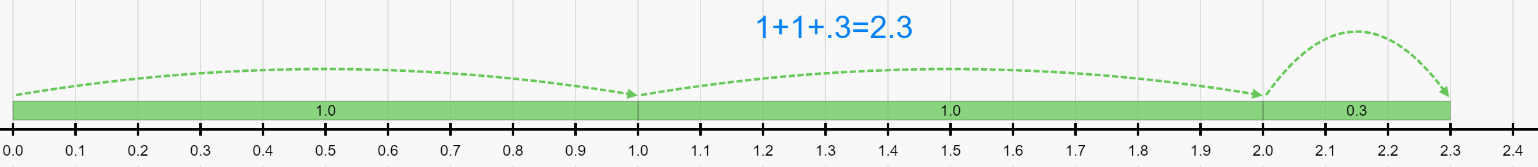# RATL 1 | Lesson 1 | Explore (Solutions: Computation on a Number Line)

## Computation on a Number Line

Use a number line to find the sums for each of the following addition problems.

1. $$10+23=10 +20+3$$1. $$1+1.3=1+1+0.3$$1. $$0.8+1.7 = 0.8+0.7+1$$ (Notice that $$0.8+0.7=1.5$$)Number lines created at https://apps.mathlearningcenter.org/number-line/